## Wednesday, November 30, 2011

### 1226

1226 = 2 x 613.

1226 has a representation as a sum of two squares: 1226 = 12 + 352.

1226 is 14401 in base 5 and 3401 in base 7.

1226 is a divisor of 653 - 1.

1226 is a central polygonal number (lazy caterer's sequence), or the maximum number of pieces formed when slicing a pancake with 49 cuts.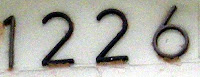The Stone Circles of Senegambia is site 1226 on the UNESCO World Heritage list.

## Tuesday, November 29, 2011

### 147

147 = 3 x 72.

147 is the number of one-sided 6-polyhexes.

147 = 142 - 72 = (14 + 7)7.

147(14 + 7) = 143 + 73 = 3087.

147 is 300 in base 7, 77 in base 20, and 70 in base 21. It is 2103 in base 4, 403 in base 6, and 103 in base 12 (and 93 in base 16, 83 in base 18, and 63 in base 24).

The binary form of 147 (10010011) contains all two-digit binary numbers (00, 01, 10, and 11) in a sequence.The digits forming 147 also form the left-hand column of a normal decimal keypad.

## Monday, November 28, 2011

### 619

619 is a prime number.

617 and 619 form a twin prime pair.

619 = 6! - 5! + 4! - 3! + 2! - 1!

619 is the only strobogrammatic prime with distinct digits. It is the smallest strobogrammatic prime that is not a palindrome.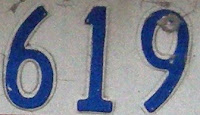Nova 619 is a webcomic.

## Wednesday, November 23, 2011

### 834

834 = 2 x 3 x 139.

834 is a divisor of 436 - 1.

834 is a cake number. 834 is the maximum number of regions into which 17 cuts can divide a cube.

834 is the sum of six consecutive primes: 834 = 127 + 131 + 137 + 139 + 149 + 151.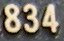The Volvo PV834 was one of a series of commercial vehicles, including ambulances, produced in the 1950s.

## Tuesday, November 22, 2011

### 132

132 = 22 x 3 x 11.

The sum of all two-digit numbers that you can make from 132 is 132: 12 + 13 + 21 + 23 + 31 + 32 = 132. It is the smallest number with this property.

132 is the sixth Catalan number.

132 is a divisor of 232 - 1.The game board for Indian snakes and ladders (Moksha Patamu) has 132 squares.

## Monday, November 21, 2011

### 225

225 = 32 x 52.

225 = 152. 225 is the smallest square that starts with two identical digits.

225 is the smallest square whose external digits (25) form a square, and whose internal digits (2) form a prime.

225 is the only three-digit square with all prime digits.

225 has a representation as a sum of two squares: 225 = 92 + 122.

225 is a divisor of 262 - 1.

225 is an octagonal number. It is also a centered octagonal number.A Scrabble board has 225 squares.

## Friday, November 18, 2011

### 928

928 = 25 x 29.

928 has a representation as a sum of two squares: 928 = 122 + 282.

928 is the sum of four consecutive primes: 928 = 227 + 229 + 233 + 239. It is also the sum of eight consecutive primes: 928 = 101 + 103 + 107 + 109 + 113 + 127 + 131 + 137.

928 is the number of ways to toss a coin 10 times and not get a run of six.The Porsche 928 was a sports car produced from 1978 to 1995.

Source: Wikipedia

## Thursday, November 17, 2011

### 1068

1068 = 22 x 3 x 89.

1068 has the representation 1068 = 210 + 44.

1068 is a divisor of 554 - 1.NGC 1068 (also known as Messier 77) is a barred spiral galaxy about 47 million light-years away in the constellation Cetus.

## Wednesday, November 16, 2011

### 309

309 = 3 x 103.

309 is a centered hendecagonal number.

309 is the smallest number whose fifth power (3095 = 2817036000549) is pandigital (contains every digit from 0 to 9 at least once).

3093 + 3090 + 3099 is a prime, with prime digital length.

309 is 373 in base 9. It is 621 in base 7 and 261 in base 11.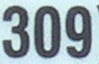309 is a telephone area code serving the state of Illinois.

Source: Number Gossip

## Tuesday, November 15, 2011

### 515

515 = 5 x 103.

515 is the number of graphs on six vertices with no isolated vertices.

515 is 20003 in base 4 and 1003 in base 8. It is 203 in base 16.

515 is the sum of nine consecutive primes: 515 = 41 + 43 + 47 + 53 + 59 + 61 + 67 + 71 + 73.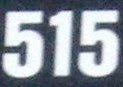In The Divine Comedy, Dante Alighieri wrote that the Apocalypse would be preceded by a messenger represented by the number "five hundred and ten and five" (515).

## Monday, November 14, 2011

### 904

904 = 23 x 113.

904 has a representation as a sum of two squares: 904 = 22 + 302.

904 is the maximum number of regions into which 42 lines divide the plane. It is a lazy caterer's number (central polygonal number) for 42 cuts.

904 has a cube that is the sum of three positive cubes.

904 is 12104 in base 5 and 4104 in base 6. It is 1214 in base 9, and it is 404 in base 15 and 323 in base 17.The Porsche 904 was an automobile produced in 1964 and 1965.

## Friday, November 11, 2011

### 111111

111111 = 3 x 7 x 11 x 13 x 37.

111111 is a divisor of 1003 - 1.

111111 has the representation 22 x 34 x 73 - 21.

The 111,111th triangular number is palindromic: 6,172,882,716. The 1111th triangular number (617,716) and the 11th triangular number (66) are also palindromic.

111111 x 111111 = 12345654321.

1 x 9 + 2 = 11
12 x 9 + 3 = 111
123 x 9 + 4 = 1111
1234 x 9 + 5 = 11111
12345 x 9 + 6 = 111111

3 x 37 = 111
33 x 3367 = 111111
3333 x 33336667 = 111111111111Source: Prime Curios!

## Thursday, November 10, 2011

### 973

973 = 7 x 139.

973 is 12343 in base 5. It is 4301 in base 6, 1301 in base 9, and 301 in base 18.

973 is the sum of five positive fifth powers: 973 = 35 + 35 + 35 + 35 + 15.

973 is divisible by the sum of the squares of its digits: 92 + 72 + 32 = 139.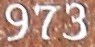Mr. 973 is a hip hop performer based in New Jersey.

## Wednesday, November 9, 2011

### 567

567 = 34 x 7.

567 is the smallest possible sum of primes that are formed using all 10 digits: 567 = 401 + 89 + 67 + 5 + 3 + 2.

567 is the smallest number that together with its square (5672 = 321489) uses the digits 1 to 9 exactly once.

567 is 4232 in base 5 and 2343 in base 6. It is 700 in base 9.

567 is a divisor of 266 - 1.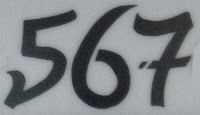The EMD 567 is a line a diesel engines produced by General Motors from 1938 until 1966 for locomotives.

## Tuesday, November 8, 2011

### 968

968 = 23 x 112.

968 is an Achilles number. Each prime factor occurs with an exponent greater than 1, but the number itself is not a perfect power.

968 has a representation as a sum of two squares: 968 = 222 + 222.

968 is a divisor of 95 - 1.

968 is 2552 in base 7 and 242 in base 21. It is 800 in base 11 and 200 in base 22.The Porsche 968 was a sports car sold from 1992 to 1995.

## Monday, November 7, 2011

### 564

564 = 22 x 3 x 47.

564 is the largest number that can be written with all the digits from 1 to 5 (without repetition) as a sum of primes in two ways: 564 = 541 + 23 = 523 + 41.

The largest prime factor of F11 (the largest completely factored Fermat number) has 564 digits.

564 is 202220 in base 3 and 4224 in base 5. It is 1434 in base 7 and 686 in base 9. It is 345 in base 13 and 234 in base 16.Interstate 564 (I-564), known as Admiral Taussig Boulevard, runs east for 3.03 miles, providing access to Naval Station Norfolk in Virginia.

## Friday, November 4, 2011

### 779

779 = 19 x 41.

779 is a divisor of 833 - 1.

779 is the sum of 11 consecutive primes: 779 = 47 + 53 + 59 + 61 + 67 + 71 + 73 + 79 + 83 + + 89 + 97.

The sum of the digits of 779 equals the sum of the digits of the 779th prime (5927): 7 + 7 + 9 = 5 + 9 + 2 + 7 = 23.

779 yields a prime when 3 is either prefixed or appended: 3779 and 7793 are both primes.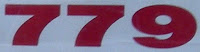Polaroid 779 was a high-speed color film for instant cameras.

## Thursday, November 3, 2011

### 559

559 = 13 x 43.

559 is 202201 in base 3 and 20233 in base 4.

559 is the sum of five consecutive primes and seven consecutive primes: 559 = 103 + 107 + 109 + 113 + 127 = 67 + 71 + 73 + 79 + 83 + 89 + 97.The German submarine U-559 sank in the Mediterranean Sea in 1942, but not before British sailors managed to recover key cryptographic material from the vessel.

## Wednesday, November 2, 2011

### 643

643 is a prime number.

641 and 643 form a twin prime pair.

643 is the largest prime factor of 123456: 643(643) = 123456.

643 = (38 + 83)/(3 + 8).A record 643 millimeters of rain fell in Sydney, Australia, in the month of June 1950.

## Tuesday, November 1, 2011

### 345

345 = 3 x 5 x 23.

345 * 2345 - 345 - 2345 is a prime number.

345 is 1333 in base 6 and 113 in base 18.

345 is the sum of three positive cubes: 345 = 73 + 13 + 13.345 is the local telephone area code for the Cayman Islands.